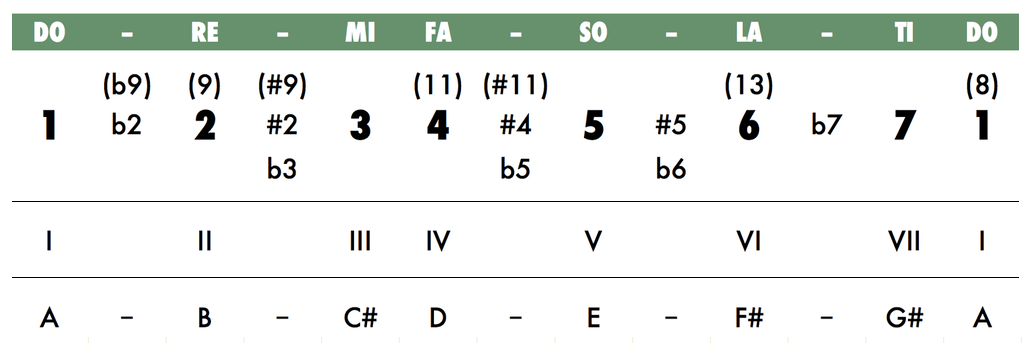Scales

GDAE - All Scales

The chart below shows the relationship between the major scale (DO-RE-MI-FA-SO-LA-TI-DO), and chromatic scale, which divides the octaves into twelve half-steps. By using a number for each tone of the major scale, (DO=1, RE=2, MI=3, etc.) a universal key of numbers is created, with which musical relationships learned in one key can be applied to all keys.

For example, the C major scale (C-D-E-F-G-A-B-C) and the A major scale (A-B-C#-D-E-F#-G#-A) can be written as 1-2-3-4-5-6-7-1. Non-major tones can also be written as numbers (Eb in the key of A would be b5).

Tones that are second octave extentions are listed in parentheses. Equivalent tones in the first octave can be found by subtracting seven (since 8=1, then 9=2, #11=#4, etc.).